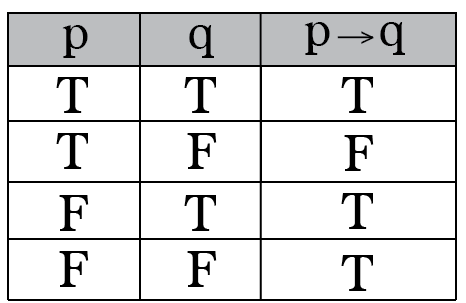Q

# I have a doubt, kindly clarify. - Mathematical reasoning - JEE Main

If   is false, then the truth values of p, q and r are, respectively :

• Option 1)

F,T,F

• Option 2)

T,F,T

• Option 3)

T,T,T

• Option 4)

F,F,F

108 Views

As we learned

Truth Table of "if-then" --for  to be 0

pqr must be (TFT) or (TFF)

Option 1)

F,T,F

Option 2)

T,F,T

Option 3)

T,T,T

Option 4)

F,F,F

Exams
Articles
Questions